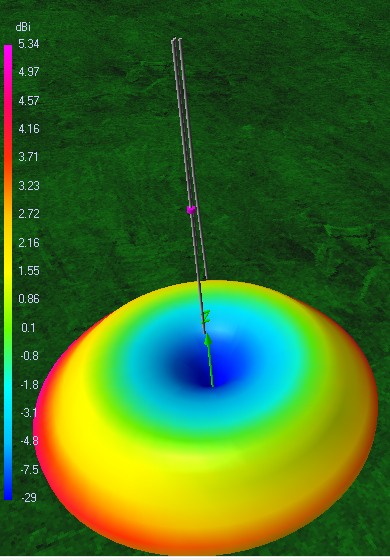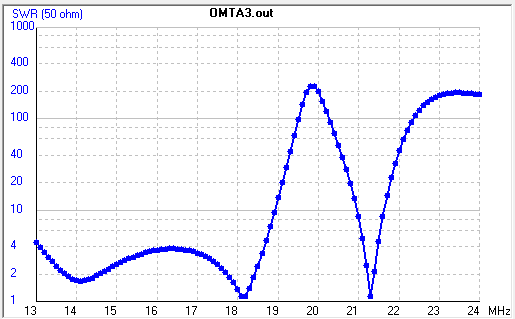OMTA stands for the offset multiband trapless antenna and was designet by Robert Wilson - VA1AKK / AL7KK and awarded in 1995 with the ARRL price for engineering originality. There are hundreds antenna of the OMTA kind around the world. It is a vertical with three bands with no traps or any other inductive loads. There are only one feedpoint of 50 Ohms and no antenna tuner is needed. It works greate almost like a single dipole. Here is my own version - The inverted OMTA which is easy to be tuned modeld in 4NEC2. Below is the source code.
CM Model : OMTA - OFFSET MULTIBAND TRAPLESS ANTENNA above ground
CM Antenna was designed by : Robert Wilson - VA1AKK / AL7KK
CM This antenna won awards for engineering originality in 1995 (see ARRL https://www.arrl.org/files/file/Technology/tis/info/pdf/9510032.pdf)
CM Antenna modeling in 4NEC2 : YO5OUC - Nicolae Crisan
CM http://www.qrz.com/db/YO5OUC
CM E-mail: [email protected]
CM
CE
SY d=0.0252    'diameter of the pipe
SY h0=5    'distance from the soil
SY h1=0.05    'distance from the ground plate
SY lg=0.2    'ground plate side
SY x1=0.1
SY x2=0.1265
SY n=51
SY m=79
SY p=2*n
SY deltaL=0.1    'segment legth
SY k=69
SY n1=20
SY m1=40
SY k1=45
SY alfa=3    '3
GW    1    p    -x1    0    h0    -x1    0    h0+p*deltaL    d/2
GW    2    m1    x1    0    h0+p*deltaL    x1    0    h0+p*deltaL-m1*deltaL    d/2
GW    3    k1    0    x2    h0+p*deltaL    0    x2    h0+p*deltaL-k1*deltaL    d/2
GW    4    m-m1    x1    0    h0+p*deltaL-m1*deltaL    (m-m1)*deltaL*sin(alfa)    0    h0+p*deltaL-m1*deltaL-(m-m1)*deltaL*cos(alfa)    d/2
GW    5    k-k1    0    x2    h0+p*deltaL-k1*deltaL    0    (k-k1)*deltaL*sin(alfa)    h0+p*deltaL-k1*deltaL-(k-k1)*deltaL*cos(alfa)    d/2
GE    1
GN    2    0    0    0    3    0.0001
EK
EX    0    1    p/2    0    1    0    0
FR    0    0    0    0    21.2    0
EN